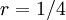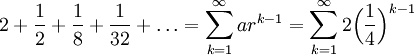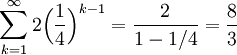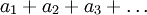# Finding the Sum of an Infinite Series

Previous: Converting an Infinite Decimal Expansion to a Rational Number

Next: A Geometric Series Problem with Shifting Indicies

## Question

Calculate the sum of the following series:## Solution

### Step (1): Express in Sigma Notation

The first four terms in the series areEach term in the series is equal to its previous multiplied by 1/4. Hence, the series is a geometric series with common ratioand first term:### Step (2): Apply Summation Formula## Explanation of Each Step

### Step (1)

In any question where one must find the sum of a series given in the formwhere each term is positive, we must first convert the sum to sigma notation. Why? Because there are no methods (covered in the ISM) to compute an infinite sum otherwise.

There are no general methods to do this, but by looking for a patterns, one might want to look for a way to relate each term by a common base. In our example here, we found that each term in the series could be related to each other with a common ratio of 1/4.

### Step (2)

Applying our summation formula with common ratioand first termyields Step (2).

## Possible Challenges

### Getting Started

The question asks us to compute the sum of an infinite series, and there are only two ways we could do this. The only two series that have methods for which we can calculate their sums are geometric and telescoping. Since each term is positive, the sum is not telescoping. So the series must be geometric, which means we can get started by looking for a common ratio,.

Previous: Converting an Infinite Decimal Expansion to a Rational Number

Next: A Geometric Series Problem with Shifting Indicies